# Introduction

This vignette documents the latest developments in hierfstat. Refer to the hierfstat article and a step by step tutorial for an introduction to the package

Data can be imported in hierfstatmany different ways (fstat format, tabular format, dosage data, even VCF format), as described in the import vignette. hierfstat can now also read genind objects (from package adegenet). Note however that only some genetic data types will be properly converted and used. The alleles need to be encoded either as integer (up to three digits per allele), or as nucleotides (c("a","c","g","t","A","C","G","T")).

library(adegenet)
library(hierfstat)
data(nancycats)
is.genind(nancycats)
##  TRUE

# Descriptive statistics

The function you are the most likely to want using is basic.stats (it calculates $$H_O$$, $$H_S$$, $$F_{IS}$$, $$F_{ST}$$ etc…).

bs.nc<-basic.stats(nancycats)
bs.nc
## $perloc ## Ho Hs Ht Dst Htp Dstp Fst Fstp Fis Dest ## fca8 0.6670 0.7790 0.8619 0.0829 0.8671 0.0881 0.0962 0.1016 0.1438 0.3987 ## fca23 0.6838 0.7439 0.7994 0.0555 0.8029 0.0589 0.0694 0.0734 0.0809 0.2302 ## fca43 0.6814 0.7442 0.7937 0.0495 0.7968 0.0526 0.0623 0.0660 0.0844 0.2054 ## fca45 0.7100 0.7085 0.7642 0.0557 0.7679 0.0594 0.0729 0.0774 -0.0021 0.2039 ## fca77 0.6295 0.7828 0.8659 0.0831 0.8711 0.0883 0.0960 0.1014 0.1958 0.4067 ## fca78 0.5773 0.6339 0.6773 0.0434 0.6801 0.0462 0.0641 0.0679 0.0893 0.1261 ## fca90 0.6454 0.7408 0.8144 0.0736 0.8190 0.0782 0.0904 0.0955 0.1287 0.3017 ## fca96 0.6259 0.6747 0.7657 0.0910 0.7714 0.0967 0.1189 0.1254 0.0723 0.2973 ## fca37 0.4485 0.5671 0.6027 0.0356 0.6049 0.0379 0.0591 0.0626 0.2091 0.0874 ## ##$overall
##     Ho     Hs     Ht    Dst    Htp   Dstp    Fst   Fstp    Fis   Dest
## 0.6299 0.7083 0.7717 0.0634 0.7757 0.0674 0.0821 0.0869 0.1108 0.2310
boxplot(bs.nc$perloc[,1:3]) # boxplot of Ho, Hs, Ht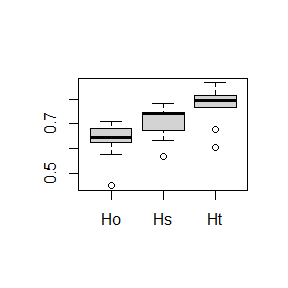You can also get e.g. allele.count and allelic.richness, a rarefied measure of the number of alleles at each locus and in each population. For instance, below is a boxplot of the allelic richness for the 5 first loci in the nancycats dataset boxplot(t(allelic.richness(nancycats)$Ar[1:5,])) #5 first loci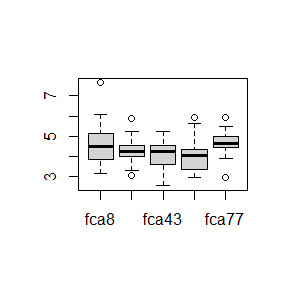# Population statistics

Population statistics are obtained through basic.stats or wc (varcomp.glob can also be used and will give the same result as wc for a one level hierarchy). For instance, $$F_{IS}$$ and $$F_{ST}$$ in the Galba truncatula dataset provided with hierfstat are obtained as:

data(gtrunchier)
wc(gtrunchier[,-2])
## $FST ##  0.540894 ## ##$FIS
##  0.8154694
varcomp.glob(data.frame(gtrunchier[,1]),gtrunchier[,-c(1:2)])$F #same ## gtrunchier...1. Ind ## Total 0.540894 0.9152809 ## gtrunchier...1. 0.000000 0.8154694 Confidence intervals on these statistics can be obtained via boot.vc: boot.vc(gtrunchier[,1],gtrunchier[,-c(1:2)])$ci
##       H-Total F-Pop/Total F-Ind/Total  H-Pop F-Ind/Pop   Hobs
## 2.5%   0.6523      0.4808      0.9045 0.2686    0.7684 0.0554
## 50%    0.7470      0.5376      0.9145 0.3418    0.8145 0.0631
## 97.5%  0.8233      0.6160      0.9269 0.4126    0.8504 0.0702

boot.ppfisand boot.ppfst provide bootstrap confidence intervals (bootstrapping over loci) for population specific $$F_{IS}$$ and pairwise $$F_{ST}$$ respectively.

# Testing

## Genetic distances

genet.dist estimates one of 10 different genetic distances between populations as described mostly in Takezaki & Nei (1996)

(Ds<-genet.dist(gtrunchier[,-2],method="Ds")) # Nei's standard genetic distances
##           1         2         3         4         5
## 2 0.4272210
## 3 1.1402899 1.8235430
## 4 0.8387367 0.9540338 1.6638485
## 5 0.6967425 0.6205417 2.5798363 0.8767008
## 6 0.9411656 0.9742812 1.1553423 0.5243353 1.1911894

## Population Principal coordinate analysis

Principal coordinate analysis can be carried out on this genetic distances:

pcoa(as.matrix(Ds))

## Population specific $$F_{ST}$$s

Function betas will give estimates of population specific $$F_{ST}^i$$ for data in the fstat format. For instance, using the nancycats dataset:

barplot(betas(nancycats)$betaiovl)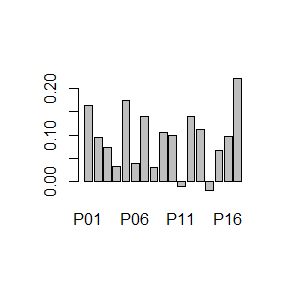Functions fs.dosage, fst.dosage and fis.dosage give estimates of population specific $$F_{ST}^i$$ and $$F_{IS}^i$$ for dosage data, fs.dosage also outputs the matrix of $$F_{ST}^{XY}$$, as defined in Weir and Goudet (2017) and Weir and Hill (2002). We use the gtrunchier dataset to illustrate the output of fs.dosage. We first need to convert gtrunchier to a dosage format via fstat2dos gt.dos<-fstat2dos(gtrunchier[,-c(1:2)]) #converts fstat format to dosage fs.gt<-fs.dosage(gt.dos,pop=gtrunchier[,2]) image(1:29,1:29,fs.gt$FsM,main=expression(F[ST]^{XY}))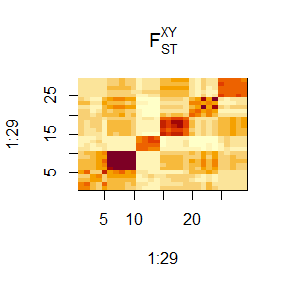We clearly see the block structure of patches within locality, with the second block along the diagonal showing higher similarity than the others. Blocks off the main diagonal are lighter, showing less genetic similarity between localities than within.

# Individual statistics

## Individual PCA

indpca carries out Principal component analysis on individual genotypes. To illustrate, we use the gtrunchier datasets, with individuals colored according to their locality of origin:

x<-indpca(gtrunchier[,-2],ind.labels=gtrunchier[,2])
plot(x,col=gtrunchier[,1],cex=0.5)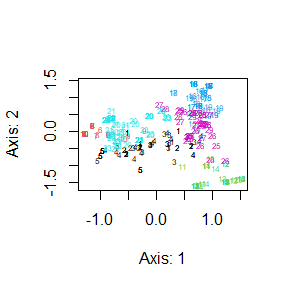## kinships, GRM and individual inbreeding coefficients

Functions betas and beta.dosage give estimates of individual inbreeding coefficients and kinships between individuals, the former for genotypes in the fstat format, the latter for dosage data:

image(beta.dosage(gt.dos),main="kinship and inbreeding \n in Galba truncatula",xlab="",ylab="")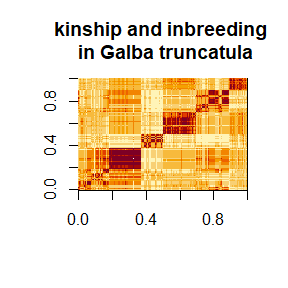This example is just for illustrating purposes, I do not advise using these individual statistics unless you have a large number of polymorphic markers ($$\geq 1000$$ at least, better if $$\geq 10000$$, see Goudet, Kay & Weir (2018)).

# Simulating genetic data

It is now possible to simulate genetic data from a continent islands model, either at equilibrium via sim.genot or for a given number of generations via sim.genot.t. These two functions have several arguments, allowing to look at the effect of population sizes, inbreeding, migration and mutation. the number of independent loci and number of alleles per loci can also be specified. It is also possible to simulate data from a finite island model using sim.genot.t, by specifying that argument IIM is FALSE. Last,sim.genot.metapop.t generates genetic data with any migration matrix, population size and inbreeding level, while still assuming independence of the genetic markers.

# Exporting to other programs

(refer to the import vignette to import data from other programs)

Other than the fstat format, hierfstatcan now export to files in format suitable for Bayescan, plink and structure. The functions to export to these programs are write.bayescan, write.ped and write.struct respectively.

# Miscellaneous

## Sex-biased dispersal

A function to detect sex-biased dispersal, sexbias.test based on Goudet et al. (2002) has been implemented. To illustrate its use, load the Crocidura russula data set. It consists of the genotypes and sexes of 140 shrews studied by Favre et al. (1997). In this species, mark -recapture showed an excess of dispersal from females, an unusual pattern in mammals. This is confirmed using genetic data:

data("crocrussula")
aic<-AIc(crocrussula$genot) boxplot(aic~crocrussula$sex)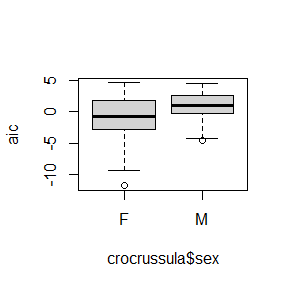tapply(aic,crocrussula$sex,mean) ## F M ## -1.1602396 0.9770438 sexbias.test(crocrussula$genot,crocrussula$sex) ##$call
## sexbias.test(dat = crocrussula$genot, sex = crocrussula$sex)
##
## $statistic ## t ## -4.117605 ## ##$p.value
##  8.097862e-05